# NCERT Solutions for Class 10 Maths Exercise 5.2## myCBSEguide App

CBSE, NCERT, JEE Main, NEET-UG, NDA, Exam Papers, Question Bank, NCERT Solutions, Exemplars, Revision Notes, Free Videos, MCQ Tests & more.

NCERT solutions for Maths Arithmetic Progression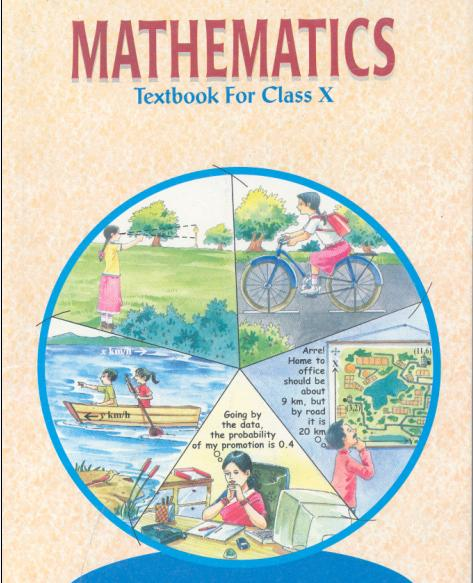## NCERT Solutions for Class 10 Maths Arithmetic Progressions

###### 1. Find the missing variable from a, d, n and an, where a is the first term, d is the common difference and an is the nth term of AP.

(i) a = 7, d = 3, n = 8

(ii) a = –18, n = 10,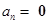(iii) d = –3, n = 18,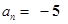(iv) a = –18.9, d = 2.5,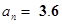(v) a = 3.5, d = 0, n = 105

Ans. (i) a = 7, d = 3, n = 8

We need to find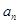here.

Using formula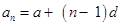Putting values of a, d and n,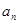= 7 + (8 − 1) 3

= 7 + (7) 3 = 7 + 21 = 28

(ii) a = –18, n= 10,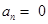We need to find d here.

Using formulaPutting values of a,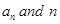,

0 = –18 + (10 – 1) d

⇒ 0 = −18 + 9d

⇒ 18 = 9d d = 2

(iii) d = –3, n = 18,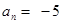We need to find a here.

Using formulaPutting values of d,,

–5 = a + (18 – 1) (–3)

⇒ −5 = a + (17) (−3)

⇒ −5 = a – 51 ⇒ a = 46

(iv) a = –18.9, d = 2.5,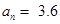We need to find n here.

Using formulaPutting values of d,,

3.6 = –18.9 + (n – 1) (2.5)

⇒ 3.6 = −18.9 + 2.5n − 2.5

⇒ 2.5n = 25 ⇒ n = 10

(v) a = 3.5, d = 0, n = 105

We need to findhere.

Using formulaPutting values of d, n and a,

an = 3.5 + (105 − 1) (0)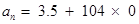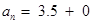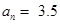NCERT Solutions for Class 10 Maths Exercise 5.2

###### 2.Choose the correct choice in the following and justify:

(i) 30th term of the AP: 10, 7, 4… is

(A) 97

(B) 77

(C) –77

(D) –87

(ii) 11th term of the AP: −3, −12, 2… is

(A) 28

(B) 22

(C) –38

(D)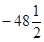Ans.(i) 10, 7, 4…

First term = a = 10, Common difference = d = 7 – 10 = 4 – 7 = –3

And n = 30{Because, we need to find 30th term}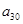= 10 + (30 − 1) (−3) = 10 – 87 = −77

(ii) −3, −½, 2…

First term = a = –3, Common difference = d = −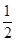− (−3) = 2 − (−) =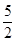And n = 11 (Because, we need to find 11th term)= −3 + (11 – 1)= −3 + 25 = 22

Therefore 11th term is 22 which means answer is (B).

NCERT Solutions for Class 10 Maths Exercise 5.2

###### 3. In the following AP’s find the missing terms:

(i) 2, __ , 26

(ii) __, 13, __, 3

(iii) 5, __, __,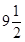(iv) –4. __, __, __, __, 6

(v) __, 38, __, __, __, –22

Ans. (i) 2, __ , 26

We know that difference between consecutive terms is equal in any A.P.

Let the missing term be x.

x – 2 = 26 – x

⇒ 2x = 28 ⇒ x = 14

Therefore, missing term is 14.

(ii)__, 13, __, 3

Let missing terms be x and y.

The sequence becomes x, 13, y, 3

We know that difference between consecutive terms is constant in any A.P.

y – 13 = 3 – y

⇒ 2y = 16 ⇒ y = 8

And 13 – x = y – 13

x + y = 26

But, we have y = 8,

x + 8 = 26⇒ x = 18

Therefore, missing terms are 18 and 8.

(iii) 5, __, __,Here, first term = a = 5 And, 4th term =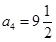Using formula, to find nth term of arithmetic progression,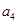= 5 + (4 − 1) d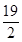= 5 + 3d

⇒ 19 = 2 (5 + 3d)

⇒ 19 = 10 + 6d

⇒ 6d = 19 – 10

⇒ 6d = 9⇒ d =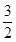Therefore, we get common difference = d =Second term = a + d =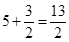Third term = second term + d =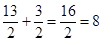Therefore, missing terms are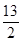and 8

(iv)–4. __, __, __, __, 6

Here, First term = a = –4 and 6th term =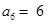Using formula, to find nth term of arithmetic progression,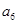= −4 + (6 − 1) d

⇒ 6 = −4 + 5d

⇒ 5d = 10 ⇒ d = 2

Therefore, common difference = d = 2

Second term = first term + d = a + d = –4 + 2 = –2

Third term = second term + d = –2 + 2 = 0

Fourth term = third term + d = 0 + 2 = 2

Fifth term = fourth term + d = 2 + 2 = 4

Therefore, missing terms are –2, 0, 2 and 4.

(v) __, 38, __, __, __, –22

We are given 2nd and 6th term.

Using formula, to find nth term of arithmetic progression,

a2 = a + (2 − 1) d And a6 = a + (6 − 1) d

⇒ 38 = a + d And −22 = a + 5d

These are equations in two variables, we can solve them using any method.

Using equation (38 = a + d), we can say that a = 38 − d.

Putting value of a in equation (−22 = a + 5d),

−22 = 38 – d + 5d

⇒ 4d = −60

d = −15

Using this value of d and putting this in equation 38 = a + d,

38 = a – 15⇒ a = 53

Therefore, we get a = 53 and d = −15

First term = a = 53

Third term = second term + d = 38 – 15 = 23

Fourth term = third term + d = 23 – 15 = 8

Fifth term = fourth term + d = 8 – 15 = –7

Therefore, missing terms are 53, 23, 8 and –7.

NCERT Solutions for Class 10 Maths Exercise 5.2

###### 4. Which term of the AP: 3, 8, 13, 18 … is 78?

Ans. First term = a = 3, Common difference = d = 8 – 3 = 13 – 8 = 5 and an = 78

Using formula, to find nth term of arithmetic progression,= 3 + (n − 1) 5,

⇒ 78 = 3 + (n − 1) 5

⇒ 75 = 5n − 5

⇒ 80 = 5nn = 16

It means 16th term of the given AP is equal to 78.

NCERT Solutions for Class 10 Maths Exercise 5.2

5. Find the number of terms in each of the following APs:

(i) 7, 13, 19…., 205

(ii) 18,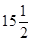, 13…, −47

Ans. (i) 7, 13, 19 …, 205

First term = a = 7, Common difference = d = 13 – 7 = 19 – 13 = 6

And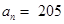Using formula, to find nth term of arithmetic progression,

205 = 7 + (n − 1) 6 = 7 + 6n – 6

⇒ 205 = 6n + 1

⇒ 204 = 6nn = 34

Therefore, there are 34 terms in the given arithmetic progression.

(ii) 18,, 13 …, −47

First term = a =18, Common difference = d =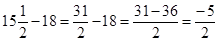And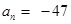Using formula, to find nth term of arithmetic progression,

−47 = 18 + (n − 1)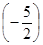= 36 −n +⇒ −94 = 36 − 5n + 5

⇒ 5n = 135 ⇒ n = 27

Therefore, there are 27 terms in the given arithmetic progression.

NCERT Solutions for Class 10 Maths Exercise 5.2

###### 6. Check whether –150 is a term of the AP: 11, 8, 5, 2…

Ans. Let −150 is the nth of AP 11, 8, 5, 2… which means that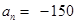Here, First term = a = 11, Common difference = d = 8 – 11 = –3

Using formula, to find nth term of arithmetic progression,

−150 = 11 + (n − 1) (−3)

⇒ −150 = 11 − 3n + 3

⇒ 3n = 164 ⇒ n =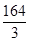But, n cannot be in fraction.

Therefore, our supposition is wrong. −150 cannot be term in AP.

NCERT Solutions for Class 10 Maths Exercise 5.2

###### 7. Find the 31st term of an AP whose 11th term is 38 and 16th term is 73.

Ans. Here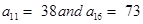Using formula, to find nth term of arithmetic progression,

38 = a + (11 − 1) (d)And73 = a + (16 − 1) (d)

⇒ 38 = a + 10d And 73 = a + 15d

These are equations consisting of two variables.

We have, 38 = a + 10d

a = 38 − 10d

Let us put value of a in equation (73 = a + 15d),

73 = 38 − 10d + 15d

⇒ 35 = 5d

Therefore, Common difference = d = 7

Putting value of d in equation 38 = a + 10d,

38 = a + 70

a = −32

Therefore, common difference = d = 7 and First term = a = –32

Using formula, to find nth term of arithmetic progression,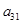= −32 + (31 − 1) (7)

= −32 + 210 = 178

Therefore, 31st term of AP is 178.

NCERT Solutions for Class 10 Maths Exercise 5.2

###### 8. An AP consists of 50 terms of which 3rd term is 12 and the last term is 106. Find the 29th term.

Ans. An AP consists of 50 terms and the 50th term is equal to 106 and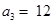Using formula, to find nth term of arithmetic progression,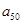= a + (50 − 1) d And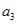= a + (3 − 1) d

⇒ 106 = a + 49d And12 = a + 2d

These are equations consisting of two variables.

Using equation 106 = a + 49d, we get a = 106 − 49d

Putting value of a in the equation 12 = a + 2d,

12 = 106 − 49d + 2d

⇒ 47d = 94 ⇒ d = 2

Putting value of d in the equation, a = 106 − 49d,

a = 106 – 49 (2) = 106 – 98 = 8

Therefore, First term = a = 8 and Common difference = d = 2

To find 29th term, we use formulawhich is used to find nth term of arithmetic progression,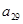= 8 + (29 − 1) 2 = 8 + 56 = 64

Therefore, 29th term of AP is equal to 64.

NCERT Solutions for Class 10 Maths Exercise 5.2

###### 9. If the third and the ninth terms of an AP are 4 and –8 respectively, which term of this AP is zero?

Ans. It is given that 3rd and 9th term of AP are 4 and –8 respectively.

It means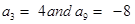Using formula, to find nth term of arithmetic progression,

4 = a + (3 – 1) d And, –8 = a + (9 – 1) d

⇒ 4 = a + 2d And, −8 = a + 8d

These are equations in two variables.

Using equation 4 = a + 2d, we can say that a = 4 − 2d

Putting value of a in other equation −8 = a + 8d,

−8 = 4 − 2d + 8d

⇒ −12 = 6d d = −2

Putting value of d in equation −8 = a + 8d,

−8 = a + 8 (−2)

⇒ −8 = a – 16 ⇒ a = 8

Therefore, first term = a = 8 and Common Difference = d = −2

We want to know which term is equal to zero.

Using formula, to find nth term of arithmetic progression,

0 = 8 + (n − 1) (−2)

⇒ 0 = 8 − 2n + 2

⇒ 0 = 10 − 2n

⇒ 2n = 10⇒ n = 5

Therefore, 5th term is equal to 0.

NCERT Solutions for Class 10 Maths Exercise 5.2

###### 10. The 17th term of an AP exceeds its 10th term by 7. Find the common difference.

Ans.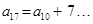(1)

Using formula, to find nth term of arithmetic progression,

a17 = a + 16d… (2)

a10 = a + 9d… (3)

Putting (2) and (3) in equation (1),

a + 16d = a + 9d + 7

⇒ 7d = 7⇒ d = 1

NCERT Solutions for Class 10 Maths Exercise 5.2

###### 11. Which term of the AP: 3, 15, 27, 39… will be 132 more than its 54th term?

Ans. Lets first calculate 54th of the given AP.

First term = a = 3, Common difference = d = 15 – 3 = 12

Using formula, to find nth term of arithmetic progression,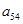= a + (54 − 1) d = 3 + 53 (12) = 3 + 636 = 639

We want to find which term is 132 more than its 54th term.

Let us suppose it is nth term which is 132 more than 54th term.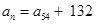⇒ 3 + (n − 1) 12 = 639 + 132

⇒ 3 + 12n – 12 = 771

⇒ 12n – 9 = 771

⇒ 12n = 780⇒ n = 65

Therefore, 65th term is 132 more than its 54th term.

NCERT Solutions for Class 10 Maths Exercise 5.2

###### 12. Two AP’s have the same common difference. The difference between their 100th terms is 100, what is the difference between their 1000th terms.

Ans. Let first term of 1st AP = a

Let first term of 2nd AP = a

It is given that their common difference is same.

Let their common difference be d.

It is given that difference between their 100th terms is 100.

Using formula, to find nth term of arithmetic progression,

a + (100 − 1) d – [a′ + (100 − 1) d]

= a + 99d a′ − 99d = 100

a a′ = 100… (1)

We want to find difference between their 1000th terms which means we want to calculate:

a + (1000 − 1) d – [a′ + (1000 − 1) d]

= a + 999d a′ − 999d = a a

Putting equation (1) in the above equation,

a + (1000 − 1) d – [a′ + (1000 − 1) d]

= a + 999d a′ + 999d = a a′ = 100

Therefore, difference between their 1000th terms would be equal to 100.

NCERT Solutions for Class 10 Maths Exercise 5.2

###### 13. How many three digit numbers are divisible by 7?

Ans. We have AP starting from 105 because it is the first three digit number divisible by 7.

AP will end at 994 because it is the last three digit number divisible by 7.

Therefore, we have AP of the form 105, 112, 119…, 994

Let 994 is the nth term of AP.

We need to find n here.

First term = a = 105, Common difference = d = 112 – 105 = 7

Using formula, to find nth term of arithmetic progression,

994 = 105 + (n − 1) (7)

⇒ 994 = 105 + 7n − 7

⇒ 896 = 7n n = 128

It means 994 is the 128th term of AP.

Therefore, there are 128 terms in AP.

NCERT Solutions for Class 10 Maths Exercise 5.2

###### 14. How many multiples of 4 lie between 10 and 250?

Ans. First multiple of 4 which lie between 10 and 250 is 12.

The last multiple of 4 which lie between 10 and 250 is 248.

Therefore, AP is of the form 12, 16, 20… ,248

First term = a = 12, Common difference = d = 4

Using formula an = a + (n – 1) d, to find nth term of arithmetic progression,

248 = 12 + (n − 1) (4)

⇒ 248 = 12 + 4n − 4

⇒ 240 = 4n n = 60

It means that 248 is the 60th term of AP.

So, we can say that there are 60 multiples of 4 which lie between 10 and 250.

NCERT Solutions for Class 10 Maths Exercise 5.2

###### 15. For what value of n, are the nth terms of two AP’s: 63, 65, 67… and 3, 10, 17… equal?

Ans. Lets first consider AP 63, 65, 67…

First term = a = 63, Common difference = d = 65 – 63 = 2

Using formula, to find nth term of arithmetic progression,

an = 63 + (n − 1) (2)… (1)

Now, consider second AP 3, 10, 17…

First term = a = 3, Common difference = d = 10 – 3 = 7

Using formula, to find nth term of arithmetic progression,

an = 3 + (n − 1) (7)… (2)

According to the given condition:

(1) = (2)

⇒ 63 + (n − 1) (2) = 3 + (n − 1) (7)

⇒ 63 + 2n – 2 = 3 + 7n − 7

⇒ 65 = 5nn = 13

Therefore, 13th terms of both the AP’s are equal.

NCERT Solutions for Class 10 Maths Exercise 5.2

###### 16. Determine the AP whose third term is 16 and the 7th term exceeds the 5th term by 12.

Ans. Let first term of AP = a

Let common difference of AP = d

It is given that its 3rd term is equal to 16.

Using formula, to find nth term of arithmetic progression,

16 = a + (3 − 1) (d)

⇒ 16 = a + 2d… (1)

It is also given that 7th term exceeds 5th term by12.

According to the given condition: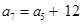a + (7 – 1) d = a + (5 – 1) d + 12

⇒ 2d = 12 ⇒ d = 6

Putting value of d in equation 16 = a + 2d,

16 = a + 2 (6) ⇒ a = 4

Therefore, first term = a = 4

And, common difference = d = 6

Therefore, AP is 4, 10, 16, 22…

NCERT Solutions for Class 10 Maths Exercise 5.2

###### 17. Find the 20th term from the last term of the AP: 3, 8, 13… , 253.

Ans. We want to find 20th term from the last term of given AP.

So, let us write given AP in this way:253 … 13, 8, 3

Here First term = a = 253, Common Difference = d = 8 – 13 = –5

Using formula, to find nth term of arithmetic progression,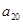= 253 + (20 − 1) (−5)= 253 + 19 (−5) = 253 – 95 = 158

Therefore, the 20th term from the last term of given AP is 158.

NCERT Solutions for Class 10 Maths Exercise 5.2

###### 18. The sum of the 4th and 8th terms of an AP is 24 and the sum of 6th and 10th terms is 44. Find the three terms of the AP.

Ans. The sum of 4th and 8th terms of an AP is 24 and sum of 6th and 10th terms is 44.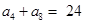and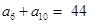Using formula, to find nth term of arithmetic progression,

a + (4 − 1) d + [a + (8 − 1) d] = 24

And, a + (6 − 1) d + [a + (10 − 1) d] = 44

a + 3d + a + 7d

= 24 And a + 5d + a + 9d = 44

⇒ 2a + 10d = 24 And 2a + 14d = 44

a + 5d = 12 And a + 7d =22

These are equations in two variables.

Using equation, a + 5d = 12, we can say that a = 12 − 5d… (1)

Putting (1) in equation a + 7d = 22,

12 − 5d + 7d = 22

⇒ 12 + 2d = 22

⇒ 2d = 10 ⇒ d = 5

Putting value of d in equation a = 12 − 5d,

a = 12 – 5 (5) = 12 – 25 = −13

Therefore, first term = a = −13 and, Common difference = d = 5

Therefore, AP is –13, –8, –3, 2…

Its first three terms are –13, –8 and –3.

NCERT Solutions for Class 10 Maths Exercise 5.2

###### 19. Subba Rao started work in 1995 at an annual salary of Rs 5000 and received an increment of Rs 200 each year. In which year did his income reach Rs 7000?

Ans. Subba Rao’s starting salary = Rs 5000

It means, first term = a = 5000

He gets an increment of Rs 200 after every year.

Therefore, common difference = d = 200

His salary after 1 year = 5000 + 200 = Rs 5200

His salary after two years = 5200 + 200 = Rs 5400

Therefore, it is an AP of the form 5000, 5200, 5400, 5600… , 7000

We want to know in which year his income reaches Rs 7000.

Using formula, to find nth term of arithmetic progression,

7000 = 5000 + (n − 1) (200)

⇒ 7000 = 5000 + 200n − 200

⇒ 7000 – 5000 + 200 = 200n

⇒ 2200 = 200n

n = 11

It means after 11 years, Subba Rao’s income would be Rs 7000.

NCERT Solutions for Class 10 Maths Exercise 5.2

###### 20. Ramkali saved Rs. 5 in the first week of a year and then increased her weekly savings by Rs. 1.75. If in the nth week, her weekly savings become Rs 20.75, find n.

Ans. Ramkali saved Rs. 5 in the first week of year. It means first term = a = 5

Ramkali increased her weekly savings by Rs 1.75.

Therefore, common difference = d = Rs 1.75

Money saved by Ramkali in the second week = a + d = 5 + 1.75 = Rs 6.75

Money saved by Ramkali in the third week = 6.75 + 1.75 = Rs 8.5

Therefore, it is an AP of the form:5, 6.75, 8.5 … , 20.75

We want to know in which year her weekly savings become 20.75.

Using formula, to find nth term of arithmetic progression,

20.75 = 5 + (n − 1) (1.75)

⇒ 20.75 = 5 + 1.75n − 1.75

⇒ 17.5 = 1.75nn = 10

It means in the 10th week her savings become Rs 20.75.

## NCERT Solutions for Class 10 Maths Exercise 5.2

NCERT Solutions Class 10 Maths PDF (Download) Free from myCBSEguide app and myCBSEguide website. Ncert solution class 10 Maths includes text book solutions from Mathematics Book. NCERT Solutions for CBSE Class 10 Maths have total 15 chapters. 10 Maths NCERT Solutions in PDF for free Download on our website. Ncert Maths class 10 solutions PDF and Maths ncert class 10 PDF solutions with latest modifications and as per the latest CBSE syllabus are only available in myCBSEguide.

## CBSE app for Class 10

To download NCERT Solutions for Class 10 Maths, Computer Science, Home Science,Hindi ,English, Social Science do check myCBSEguide app or website. myCBSEguide provides sample papers with solution, test papers for chapter-wise practice, NCERT solutions, NCERT Exemplar solutions, quick revision notes for ready reference, CBSE guess papers and CBSE important question papers. Sample Paper all are made available through the best app for CBSE students and myCBSEguide website.### 14 thoughts on “NCERT Solutions for Class 10 Maths Exercise 5.2”

1. Nice app

2. Thanks it help me in my maths homework

3. Gud bt i think in sequence all th exercises were there n then ????

4. d=5/2 kaise doondha samajh nahi aayi

5. Well done ???

6. very nice it help me in exam time

7. thank you it help me to complete my notes

8. I like this website …
Thnks

9. nice i understand well and it help in doing my homework
thank you so much
thnx

10. Thanks CBSE guide. Com

11. I like solutions

12. Well done

13. Nice hai.

14. Good one